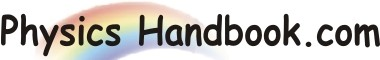HOME TOPICS DEFINITIONS TABLES LAWS INVENTIONS EXPERIMENTS QUIZ VIDEOS
 Wiedemann-Franz Law Wiedemann-Franz law states that the ratio of the thermal conductivity ( K ) to the electrical conductivity ( σ ) of a metal is proportional to the temperature ( T ). i.e. K / σ = L x T This law is named after Gustav Wiedemann and Rudolph Franz, who in 1853 reported that K / σ has approximately the same value for different metals at the same temperature. The proportionality of K / σ with temperature was discovered by Ludvig Lorenz in 1872. The proportionality constant L, known as the Lorenz number, is L = K / σ x T  =  ( π2 / 3 ) x ( kB / e )2 L  =  2.44 x 10-8 W Ω K-2 This relationship is based upon the fact that the heat and electrical transport both involve the free electrons in the metal. The thermal conductivity increases with the average particle velocity since this increases the forward transport of energy. The electrical conductivity, on the other hand, decreases while particle velocity increases because the collisions divert the electrons from forward transport of charge.